Examples

Chapter 3 Class 11 Trigonometric Functions
Serial order wise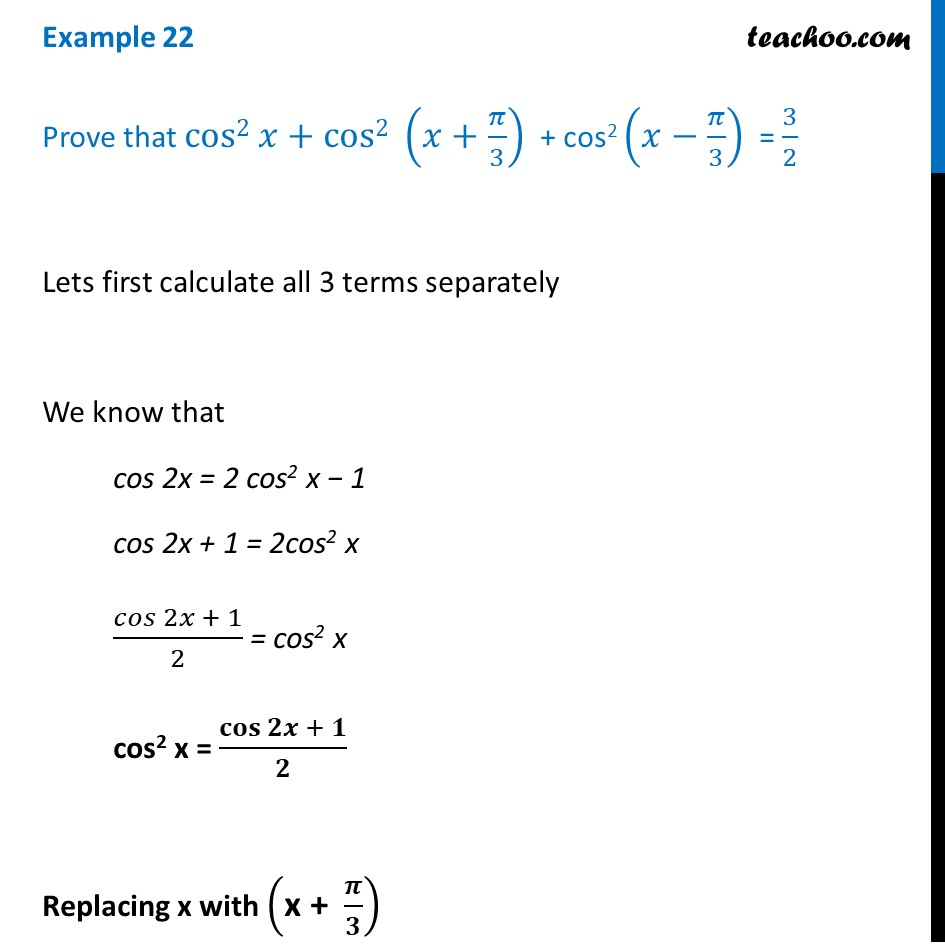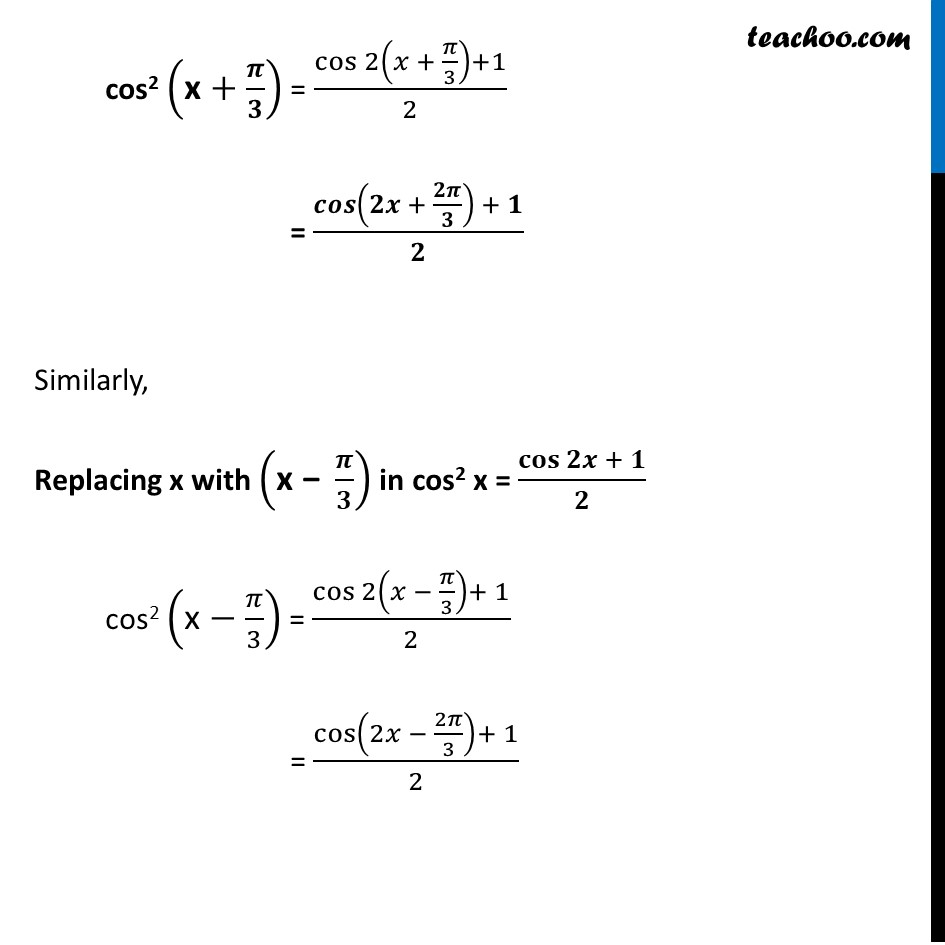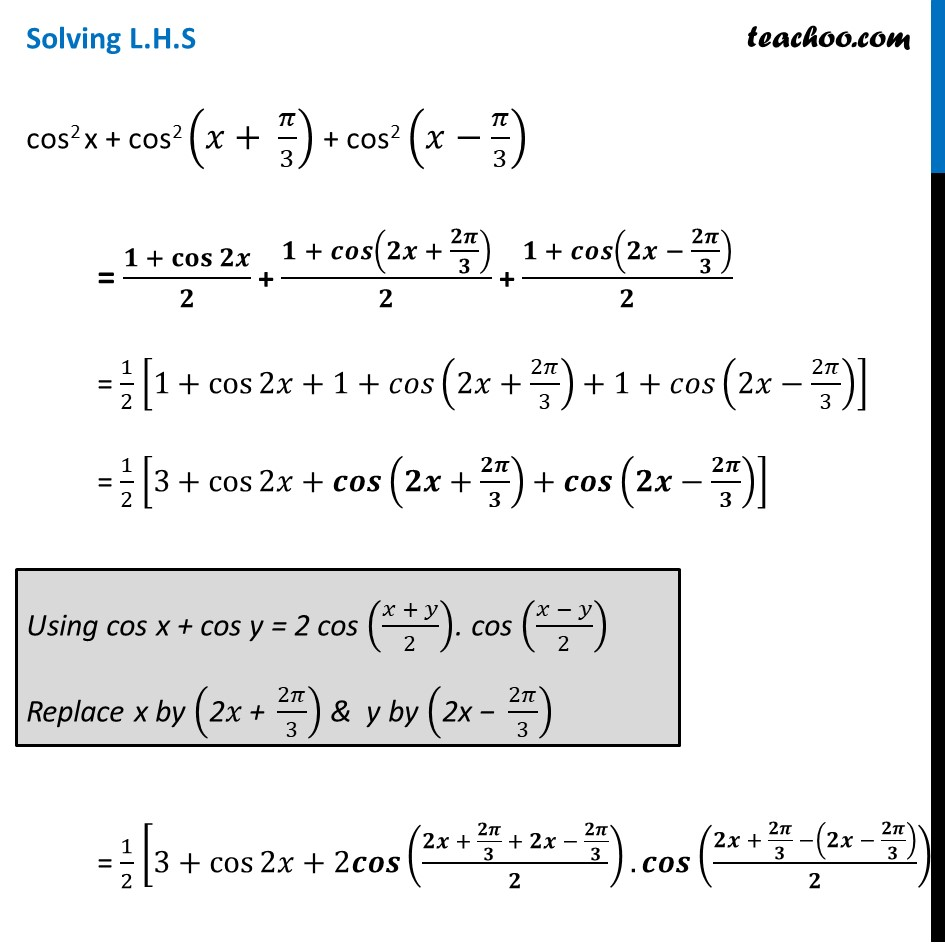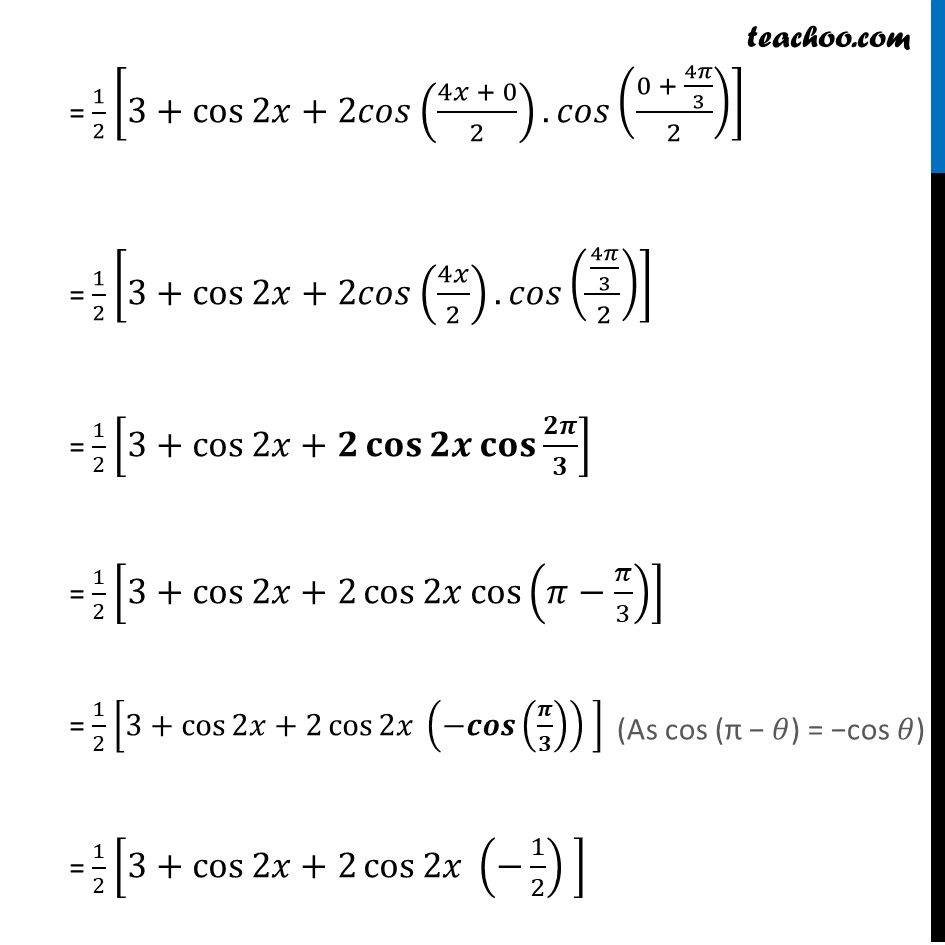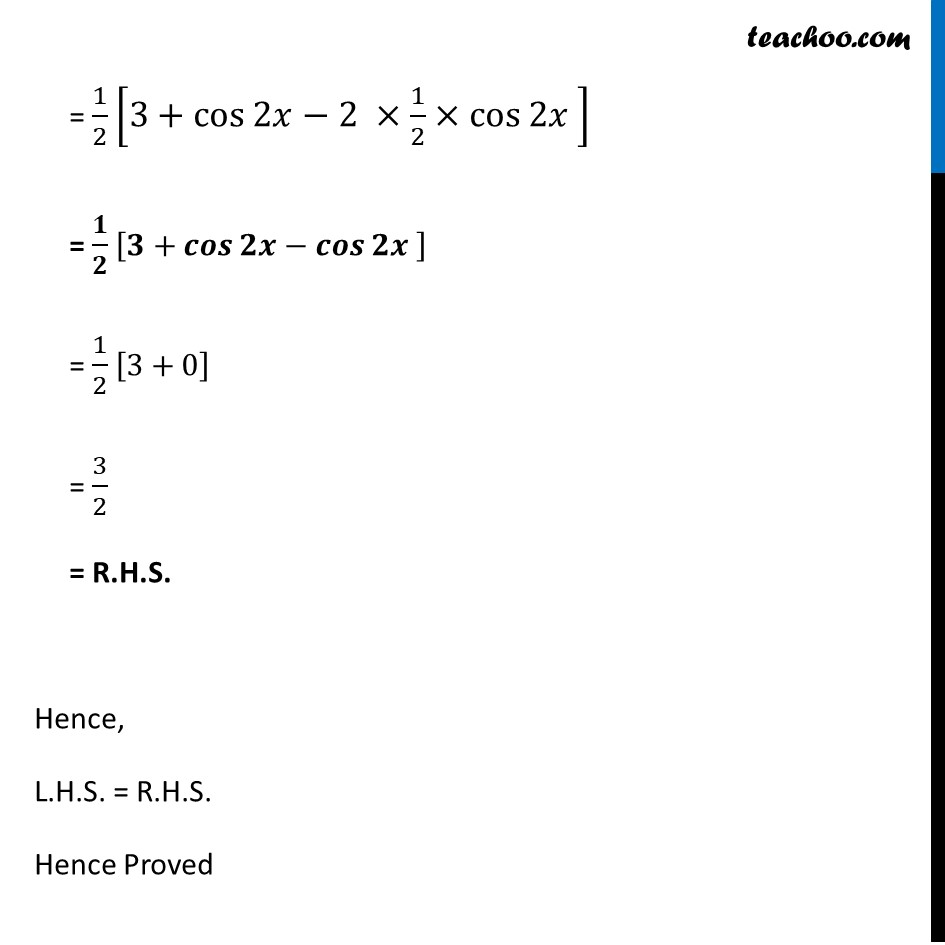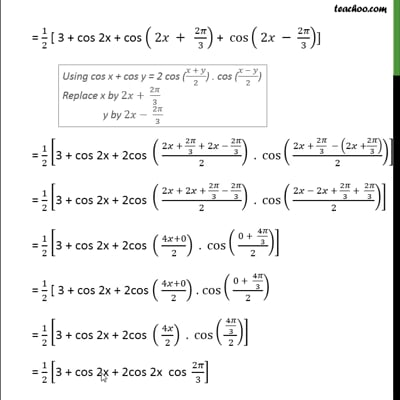This video is only available for Teachoo black users

Learn in your speed, with individual attention - Teachoo Maths 1-on-1 Class

### Transcript

Example 22 Prove that cos2 𝑥+cos2 (𝑥+𝜋/3) + cos2 (𝑥−𝜋/3) = 3/2 Lets first calculate all 3 terms separately We know that cos 2x = 2 cos2 x − 1 cos 2x + 1 = 2cos2 x 𝑐𝑜𝑠⁡〖2𝑥 + 1〗/2 = cos2 x cos2 x = 𝐜𝐨𝐬⁡〖𝟐𝒙 + 𝟏〗/𝟐 Replacing x with ("x + " 𝝅/𝟑) cos2 ("x" +𝝅/𝟑) = cos⁡〖2(𝑥 + 𝜋/3)+1〗/2 = 𝒄𝒐𝒔⁡〖(𝟐𝒙 + 𝟐𝝅/𝟑) + 𝟏〗/𝟐 Similarly, Replacing x with ("x −" 𝝅/𝟑) in cos2 x = 𝐜𝐨𝐬⁡〖𝟐𝒙 + 𝟏〗/𝟐 cos2 ("x" −𝜋/3) = cos⁡〖2(𝑥 − 𝜋/3)+ 1〗/2 = cos⁡〖(2𝑥 − 2𝜋/3)+ 1〗/2 Solving L.H.S cos2 x + cos2 (𝑥+ 𝜋/3) + cos2 (𝑥−𝜋/3) = (𝟏 + 𝐜𝐨𝐬⁡𝟐𝒙)/𝟐 + (𝟏 + 𝒄𝒐𝒔⁡(𝟐𝒙 + 𝟐𝝅/𝟑))/𝟐 + (𝟏 + 𝒄𝒐𝒔⁡(𝟐𝒙 − 𝟐𝝅/𝟑))/𝟐 = 1/2 [1+cos⁡〖2𝑥+1+𝑐𝑜𝑠(2𝑥+2𝜋/3)+1+𝑐𝑜𝑠(2𝑥−2𝜋/3)〗 ] = 1/2 [3+cos⁡〖2𝑥+𝒄𝒐𝒔(𝟐𝒙+𝟐𝝅/𝟑)+𝒄𝒐𝒔(𝟐𝒙−𝟐𝝅/𝟑)〗 ] = 1/2 [3+cos⁡〖2𝑥+2𝒄𝒐𝒔((𝟐𝒙 + 𝟐𝝅/𝟑 + 𝟐𝒙 − 𝟐𝝅/𝟑)/𝟐).𝒄𝒐𝒔((𝟐𝒙 + 𝟐𝝅/𝟑 −(𝟐𝒙 − 𝟐𝝅/𝟑))/𝟐)〗 ] = 1/2 [3+cos⁡〖2𝑥+2𝑐𝑜𝑠((4𝑥 + 0)/2).𝑐𝑜𝑠((0 + 4𝜋/3)/2)〗 ] = 1/2 [3+cos⁡〖2𝑥+2𝑐𝑜𝑠(4𝑥/2).𝑐𝑜𝑠((4𝜋/3)/2)〗 ] = 1/2 [3+cos⁡〖2𝑥+𝟐 𝐜𝐨𝐬⁡𝟐𝒙 𝐜𝐨𝐬⁡〖𝟐𝝅/𝟑〗 〗 ] = 1/2 [3+cos⁡〖2𝑥+2 cos⁡2𝑥 cos⁡(𝜋−𝜋/3) 〗 ] = 1/2 [3+cos⁡〖2𝑥+2 cos⁡2𝑥 〗 (〖−𝒄𝒐𝒔〗⁡(𝝅/𝟑) ) ] = 1/2 [3+cos⁡〖2𝑥+2 cos⁡2𝑥 〗 (−1/2) ] = 1/2 [3+cos⁡〖2𝑥−2 ×1/2×cos⁡2𝑥 〗 ] = 𝟏/𝟐 [𝟑+𝒄𝒐𝒔⁡〖𝟐𝒙−𝒄𝒐𝒔⁡𝟐𝒙 〗 ] = 1/2 [3+0] = 3/2 = R.H.S. Hence, L.H.S. = R.H.S. Hence Proved